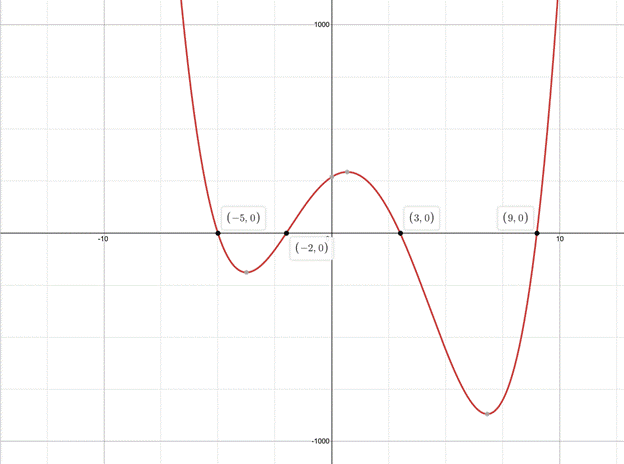# CAT 2021 Question Paper | Quant Slot 1

###### CAT Previous Year Paper | CAT Quant Questions | Question 1

CAT 2021 Quant was dominated by Arithmetic followed by Algebra. In Arithmetic, the questions were dominated by topics like Speed-time-distance, Mixture and Alligations. This year, there was a surprise. The questions from Geometry were relatively on the lower side as compared to the previous years. There were 8 TITA Qs this year. Overall this section was at a medium level of difficulty.

Question 1 : f(x) = $$frac{x^{2}+2 x-15}{x^{2}-7 x-18}$ is negative if and only if 1. -5 < x < -2 or 3 < x < 9 2. -2 < x < 3 or x > 9 3. x < -5 or 3 < x < 9 4. x < -5 or -2 < x < 3 ## Rs. 4000 Price Hike Incoming! Grab our CAT courses now at a lower price Valid until 3rd April #### 2IIM : Best Online CAT Coaching. ### Video Explanation ## Best CAT Coaching in Chennai #### CAT Coaching in Chennai - CAT 2022Limited Seats Available - Register Now! ### Explanatory Answer f$x) = $$frac{x^{2}+2 x-15}{x^{2}-7 x-18}$ f$x) = $$frac{$x+5$(x-3)}{(x-9)(x+2)})
-5, -2, 3, 9 are the roots of the function f(x).Keeping these roots on the number line and checking for the range where f(x) becomes negative.
For the numbers below -5, f(x) is positive.
For the numbers between -5 and -2, the value f(x) is negative.
For the numbers between -2 and 3, the value of f(x) is positive.
For the numbers between 3 and 9, the value of f(x) is negative.
For the numbers above 9, the value of f(x) is positive.
Hence the range should be -5 < x < -2 and 3 < x < 9.

The question is " f(x) = $$frac{x^{2}+2 x-15}{x^{2}-7 x-18}$ is negative if and only if " ##### Hence, the answer is '-5 < x < -2 or 3 < x < 9' Choice A is the correct answer. ###### Rs. 4000 Price Hike Incoming! Grab our CAT courses now at a lower price. Valid until 3rd April ###### Prepare for CAT 2023 with 2IIM's Daily Preparation Schedule ###### Know all about CAT Exam Syllabus and what to expect in CAT ###### Best CAT Online Coaching Try upto 40 hours for free Learn from the best! ###### Already have an Account? ###### CAT Coaching in ChennaiCAT 2023 Classroom Batches Starting Now! @Gopalapuram ###### Best CAT Coaching in Chennai Introductory offer of 5000/- Attend a Demo Class ###### Best Indore IPM & Rohtak IPM CoachingSignup and sample 9 full classes for free. Register now! ##### Where is 2IIM located? 2IIM Online CAT Coaching A Fermat Education Initiative, 58/16, Indira Gandhi Street, Kaveri Rangan Nagar, Saligramam, Chennai 600 093 ##### How to reach 2IIM? Mobile:$91) 99626 48484 / 94459 38484
WhatsApp: WhatsApp Now
Email: info@2iim.com